# 3: Describing Data, Part 2

3: Describing Data, Part 2

## Objectives

Upon successful completion of this lesson, you will be able to:

• Construct and interpret a boxplot and side-by-side boxplots
• Use the IQR method to identify outliers
• Construct and interpret histograms with groups and dotplots with groups
• Construct and interpret a scatterplot
• Compute and interpret a correlation
• Construct and interpret a simple linear regression model (i.e., one explanatory and one response variable)
• Compute and interpret residuals from a simple linear regression model
• Interpret plots of more than two variables
• Select an appropriate graphical representation for a given scenario

* All graph construction and computations in this lesson will be conducted in Minitab

This lesson corresponds to Sections 2.4, 2.5, 2.6, and 2.7 in the Lock5 textbook. This week you will be learning how to describe and display data concerning the relationship between one categorical and one quantitative variable or the relationship between two quantitative variables.

In our discussion of the relationship between two quantitative variables it is assumed that you have a basic understanding of linear equations (i.e., $$y=mx+b$$). For a review of basic linear equations, see Lesson 0.1.4 of the online notes.

# 3.1 - Single Boxplot

3.1 - Single Boxplot

At the end of Lesson 2.2.10 you learned that the five-number summary includes five values: minimum, Q1, median, Q3, and maximum. These five values can be used to construct a graph known as a boxplot. This is sometimes also referred to as a box and whisker plot.

In a boxplot, outliers are denoted with an asterisk. The bottom whisker extends to the lowest value that is not an outlier and the upper whisker extends to the highest value that is not an outlier. The box represents the middle 50% of observations with the lower end of the box at the 25th percentile (i.e., Q1) and the upper end of the box at the 75th percentile (i.e., Q3). The line in the middle of the box represents the median.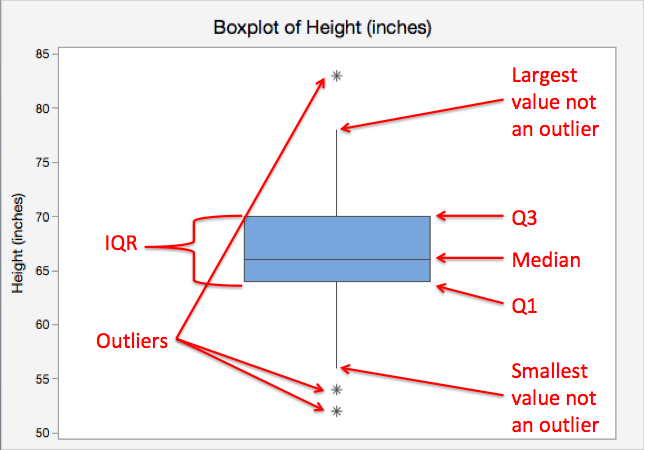Single Boxplot
Graph displaying data from one quantitative variable. Also known as a "box-and-whisker plot." The box represents the middle 50% of observed values. The bottom of the box is the first quartile (25th percentile) and the top of the box is the third quartile (75th percentile). The line in the middle of the box is the median (50th percentile). The lines, also known as whiskers, extend to the lowest and highest values that are not outliers. Outliers are symbolized using asterisks or circles.

## Minitab® – Single Boxplot

### Boxplots

This example will use data collected from a sample of students enrolled in online sections of STAT 200 during the Summer 2020 semester. These data can be downloaded as a CSV file:

To create a boxplot of the number of online courses completed:

1. Open the data set in Minitab
2. From the tool bar, select Graph > Boxplot...
3. Under One Y Variable, select Simple
4. Click OK
5. Double click the variable Online Courses Completed in the box on the left to insert it into the Y-variable box on the right
6. Click OK

This should result in the following boxplot:

Video Walkthrough

# 3.2 - Identifying Outliers: IQR Method

3.2 - Identifying Outliers: IQR Method

Some observations within a set of data may fall outside the general scope of the other observations. Such observations are called outliers. In Lesson 2.2.2 you identified outliers by looking at a histogram or dotplot. Here, you will learn a more objective method for identifying outliers.

We can use the IQR method of identifying outliers to set up a “fence” outside of Q1 and Q3. Any values that fall outside of this fence are considered outliers. To build this fence we take 1.5 times the IQR and then subtract this value from Q1 and add this value to Q3. This gives us the minimum and maximum fence posts that we compare each observation to. Any observations that are more than 1.5 IQR below Q1 or more than 1.5 IQR above Q3 are considered outliers. This is the method that Minitab uses to identify outliers by default.

## Example: Test Scores

A teacher wants to examine students’ test scores. Their scores are: 74, 88, 78, 90, 94, 90, 84, 90, 98, and 80.

Five number summary: 74, 80, 89, 90, 98.

$$IQR = 90 - 80 = 10$$

The interquartile range is 10.

$$1.5 IQR = 1.5 (10) = 15$$

1.5 times the interquartile range is 15. Our fences will be 15 points below Q1 and 15 points above Q3.

Lower fence: $$80 - 15 = 65$$
Upper fence: $$90 + 15 = 105$$

Any scores that are less than 65 or greater than 105 are outliers. In this case, there are no outliers.

## Example: BooksA survey was given to a random sample of 20 sophomore college students. They were asked, “how many textbooks do you own?” Their responses, were: 0, 0, 2, 5, 8, 8, 8, 9, 9, 10, 10, 10, 11, 12, 12, 12, 14, 15, 20, and 25.

The observations are in order from smallest to largest, we can now compute the IQR by finding the median followed by Q1 and Q3.

$$Median = 10$$

$$Q1 = 8$$

$$Q3 = 12$$

$$IQR = 12 - 8 = 4$$

The interquartile range is 4.

$$1.5 IQR = 1.5 (4) = 6$$

1.5 times the interquartile range is 6. Our fences will be 6 points below Q1 and 6 points above Q3.

Lower fence: $$8 - 6 = 2$$
Upper fence: $$12 + 6 = 18$$

Any observations less than 2 books or greater than 18 books are outliers. There are 4 outliers: 0, 0, 20, and 25.

# 3.3 - One Quantitative and One Categorical Variable

3.3 - One Quantitative and One Categorical Variable

Often times we want to compare groups in terms of a quantitative variable.  For example, we may want to compare the heights of males and females. In this case height is a quantitate variable while biological sex is a categorical variable. Graphs with groups can be used to compare the distributions of heights in these two groups. Below you will find examples of constructing side-by-side boxplots, dotplots with groups, and histograms with groups using Minitab. The side-by-side boxplots allow us to easily compare the median, IQR, and range of the two groups. The dotplots with groups and histograms with groups allow us to compare the shape, central tendency, and variability of the two groups.

## Side-by-Side Boxplots

This example will use data collected from a sample of students enrolled in online sections of STAT 200.

To create a side-by-side boxplots of Online Courses Completed by Primary Campus in Minitab:

1. Open the data file in Minitab
2. Select Graph > Boxplot... > One Y Variable > With Categorical Groups
3. Double click the variable Online Courses Completed in the box on the left to insert it into the Y variable box on the right
4. Double click the variable Campus in the box on the left to insert it into the Group variable box on the right
5. Click OK

This should result in the following side-by-side boxplots:

Video Walkthrough

## Minitab® – Dotplots with Groups

This example will use data collected from a sample of students enrolled in online sections of STAT 200.

To create a dotplot with groups in Minitab :

1. Open the data file in Minitab
2. From the tool bar, select Graph > Dotplot... > One Y Variable > Groups Displayed on the Scale
3. Double click the variable Online Courses Completed in the box on the left to insert it into the Y variable box on the right
4. Double click the variable Primary Campus in the box on the left to insert it into the Group variable box on the right
5. Click OK

This should result in the following dotplot with groups:

Video Walkthrough

## Minitab® – Histograms with Groups

This example will use data collected from a sample of students enrolled in online sections of STAT 200.

To create histograms with groups in Minitab :

1. Open the data file in Minitab
2. From the tool bar, select Graph > Histogram... > One Y Variable > Groups Displayed Separately
3. Double click the variable Online Courses Completed in the box on the left to insert it into the Y-variable box on the right
4. Double click the variable Primary Campus in the box on the left to insert it into the Group variables box on the right
5. Click OK

This should result in the histogram with groups below:

Video Walkthrough

# 3.4 - Two Quantitative Variables

3.4 - Two Quantitative Variables

Later in the course, we will devote an entire lesson to analyzing two quantitative variables. In this lesson, you will be introduced to scatterplots, correlation, and simple linear regression. A scatterplot is a graph used to display data concerning two quantitative variables. Correlation is a measure of the direction and strength of the relationship between two quantitative variables. Simple linear regression uses one quantitative variable to predict a second quantitative variable. You will not need to compute correlations or regression models by hand in this course. There is one example of computing a correlation by hand in the notes to show you how it relates to z scores, but for all assignments, you should be using Minitab to compute these statistics.

# 3.4.1 - Scatterplots

3.4.1 - Scatterplots

Recall from Lesson 1.1.2, in some research studies one variable is used to predict or explain differences in another variable. In those cases, the explanatory variable is used to predict or explain differences in the response variable.

Explanatory variable

Variable that is used to explain variability in the response variable, also known as an independent variable or predictor variable; in an experimental study, this is the variable that is manipulated by the researcher.

Response variable

The outcome variable, also known as a dependent variable.

A scatterplot can be used to display the relationship between the explanatory and response variables. Or, a scatterplot can be used to examine the association between two variables in situations where there is not a clear explanatory and response variable. For example, we may want to examine the relationship between height and weight in a sample but have no hypothesis as to which variable impacts the other; in this case, it does not matter which variable is on the x-axis and which is on the y-axis.

Scatterplot
A graphical representation of two quantitative variables in which the explanatory variable is on the x-axis and the response variable is on the y-axis.

When examining a scatterplot, we need to consider the following:

1. Direction (positive or negative)
2. Form (linear or non-linear)
3. Strength (weak, moderate, strong)
4. Bivariate outliers

In this class, we will focus on linear relationships. This occurs when the line-of-best-fit for describing the relationship between x and y is a straight line. The linear relationship between two variables is positive when both increase together; in other words, as values of x get larger values of y get larger. This is also known as a direct relationship. The linear relationship between two variables is negative when one increases as the other decreases. For example, as values of x get larger values of y get smaller. This is also known as an indirect relationship.

A bivariate outlier is an observation that does not fit with the general pattern of the other observations.

## Example: BaseballData concerning baseball statistics and salaries from the 1991 and 1992 seasons is available at:

The scatterplot below shows the relationship between salary and batting average for the 337 baseball players in this sample.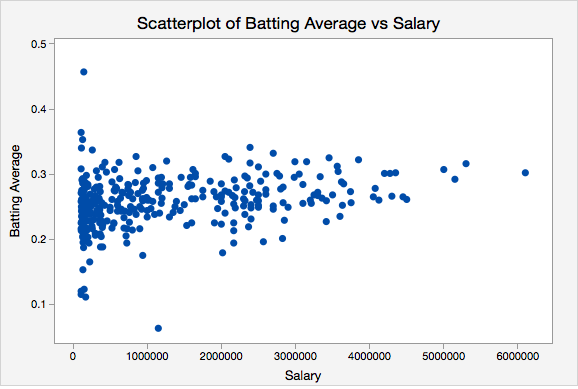From this scatterplot, we can see that there does not appear to be a meaningful relationship between baseball players' salaries and batting averages. We can also see that more players had salaries at the low end and fewer had salaries at the high end.

## Example: Height and Shoe SizeData concerning the heights and shoe sizes of 408 students were retrieved from:

The scatterplot below was constructed to show the relationship between height and shoe size.There is a positive linear relationship between height and shoe size in this sample. The magnitude of the relationship appears to be strong. There do not appear to be any outliers.

## Example: Height and Weight

The scatterplot below shows the relationship between height and weight.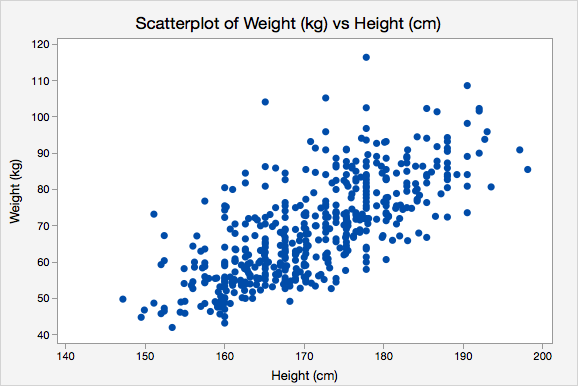There is a positive linear relationship between height and weight. The magnitude of the relationship is moderately strong.

## Example: CafésThe scatterplot below shows the relationship between maximum daily temperature and coffee sales.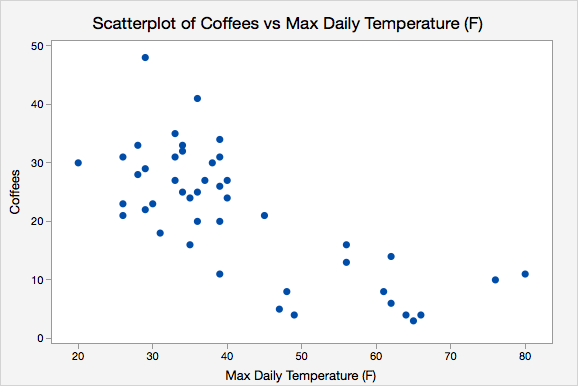There is a negative linear relationship between the maximum daily temperature and coffee sales. The magnitude is moderately strong. There do not appear to be any outliers.

# 3.4.1.1 - Minitab: Simple Scatterplot

3.4.1.1 - Minitab: Simple Scatterplot

## Minitab® – Simple Scatterplot

The file below contains data concerning students' quiz averages and final exam scores. Let's construct a scatterplot with the quiz averages on the horizontal axis and final exam scores on the vertical axis.

1. Open the data file in Minitab
2. From the tool bar, select Graphs > Scatterplot > One Y Variable > Simple
3. Double click the variable Final on the left to move it to the Y variable box on the right
4. Double click the variable Quiz_Average on the left to move it to the  X variable box on the right
5. Click OK

This should result in the scatterplot below:

Video Walkthrough

# 3.4.2 - Correlation

3.4.2 - Correlation

In this course, we will be using Pearson's $$r$$ as a measure of the linear relationship between two quantitative variables. In a sample, we use the symbol $$r$$. In a population, we use the Greek letter $$\rho$$ ("rho"). Pearson's $$r$$ can easily be computed using statistical software.

Correlation
A measure of the direction and strength of the relationship between two variables.
Properties of Pearson's r
1. $$-1\leq r \leq +1$$
2. For a positive association, $$r>0$$, for a negative association $$r<0$$, if there is no relationship $$r=0$$
3. The closer $$r$$ is to $$0$$ the weaker the relationship and the closer to $$+1$$ or $$-1$$ the stronger the relationship (e.g., $$r=-0.88$$ is a stronger relationship than $$r=+0.60$$); the sign of the correlation provides direction only
4. Correlation is unit free; the $$x$$ and $$y$$ variables do NOT need to be on the same scale (e.g., it is possible to compute the correlation between height in centimeters and weight in pounds)
5. It does not matter which variable you label as $$x$$ and which you label as $$y$$. The correlation between $$x$$ and $$y$$ is equal to the correlation between $$y$$ and $$x$$.

The following table may serve as a guideline when evaluating correlation coefficients:

Absolute Value of $$r$$ Strength of the Relationship
0 - 0.2 Very weak
0.2 - 0.4 Weak
0.4 - 0.6 Moderate
0.6 - 0.8 Strong
0.8 - 1.0 Very strong
Cautions
1. Correlation does NOT equal causation. A strong relationship between $$x$$ and $$y$$ does not necessarily mean that $$x$$ causes $$y$$. It is possible that $$y$$ causes $$x$$, or that a confounding variable causes both $$x$$ and $$y$$.
2. Pearson's $$r$$ should only be used when there is a linear relationship between $$x$$ and $$y$$. A scatterplot should be constructed before computing Pearson's $$r$$ to confirm that the relationship is not non-linear.
3. Pearson's $$r$$ is not resistant to outliers. Figure 1 below provides an example of an influential outlier. Influential outliers are points in a data set that increase the correlation coefficient. In Figure 1 the correlation between $$x$$ and $$y$$ is strong ($$r=0.979$$). In Figure 2 below, the outlier is removed. Now, the correlation between $$x$$ and $$y$$ is lower ($$r=0.576$$) and the slope is less steep.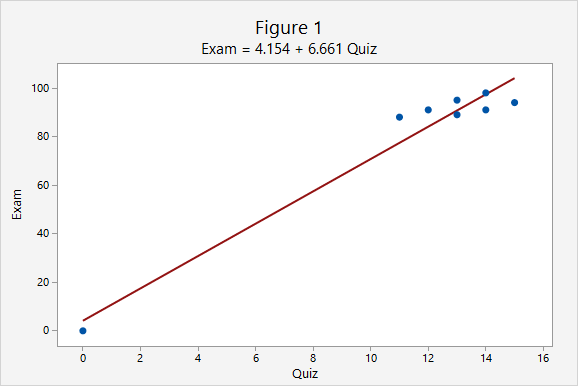Note that the scale on both the x and y axes has changed. In addition to the correlation changing, the y-intercept changed from 4.154 to 70.84 and the slope changed from 6.661 to 1.632.

# 3.4.2.1 - Formulas for Computing Pearson's r

3.4.2.1 - Formulas for Computing Pearson's r

There are a number of different versions of the formula for computing Pearson's $$r$$. You should get the same correlation value regardless of which formula you use. Note that you will not have to compute Pearson's $$r$$ by hand in this course. These formulas are presented here to help you understand what the value means. You should always be using technology to compute this value.

First, we'll look at the conceptual formula which uses $$z$$ scores. To use this formula we would first compute the  $$z$$ score for every $$x$$ and $$y$$ value. We would multiply each case's $$z_x$$ by their $$z_y$$.  If their  $$x$$ and  $$y$$ values were both above the mean then this product would be positive. If their x and y values were both below the mean this product would be positive. If one value was above the mean and the other was below the mean this product would be negative. Think of how this relates to the correlation being positive or negative. The sum of all of these products is divided by $$n-1$$ to obtain the correlation.

Pearson's r: Conceptual Formula

$$r=\dfrac{\sum{z_x z_y}}{n-1}$$
where $$z_x=\dfrac{x - \overline{x}}{s_x}$$ and $$z_y=\dfrac{y - \overline{y}}{s_y}$$

When we replace $$z_x$$ and $$z_y$$ with the $$z$$ score formulas and move the $$n-1$$ to a separate fraction we get the formula in your textbook: $$r=\frac{1}{n-1}\Sigma{\left(\frac{x-\overline x}{s_x}\right) \left( \frac{y-\overline y}{s_y}\right)}$$

# 3.4.2.2 - Example of Computing r by Hand (Optional)

3.4.2.2 - Example of Computing r by Hand (Optional)

Again, you will not need to compute $$r$$ by hand in this course. This example is meant to show you how $$r$$ is computed with the intention of enhancing your understanding of its meaning. In this course, you will always be using Minitab or StatKey to compute correlations.

In this example we have data from a random sample of $$n = 9$$ World Campus STAT 200 students from the Spring 2017 semester. WileyPlus scores had a maximum possible value of 100. Midterm exam scores had a maximum possible value of 50. Remember, the $$x$$ and $$y$$ variables do not need to be on the same metric to compute a correlation.

ID WileyPlus Midterm
A 82 37
B 100 47
C 96 33
D 96 36
E 80 44
F 77 35
G 100 50
H 100 49
I 94 45

Minitab was used to construct a scatterplot of these two variables. We need to examine the shape of the relationship before determining if Pearson's $$r$$ is the appropriate correlation coefficient to use. Pearson's $$r$$ can only be used to check for a linear relationship. For this example I am going to call WileyPlus grades the $$x$$ variable and midterm exam grades the $$y$$ variable because students completed WileyPlus assignments before the midterm exam.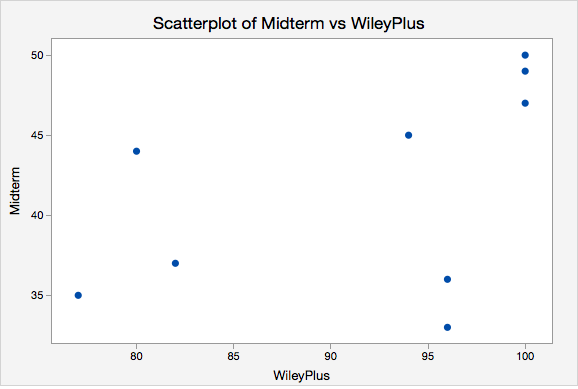### Summary Statistics

From this scatterplot we can determine that the relationship may be weak, but that it is reasonable to consider a linear relationship. If we were to draw a line of best fit through this scatterplot we would draw a straight line with a slight upward slope. Now, we'll compute Pearson's $$r$$ using the $$z$$ score formula. The first step is to convert every WileyPlus score to a $$z$$ score and every midterm score to a $$z$$ score. When we constructed the scatterplot in Minitab we were also provided with summary statistics including the mean and standard deviation for each variable which we need to compute the $$z$$ scores.

Statistics
Variable N* Mean StDev Minimum Maximum
Midterm 9 41.778 6.534 33.000 50.000
WileyPlus 9 91.667 9.327 77.000 100.000
ID WileyPlus $$z_x$$
A 82 $$\frac{82-91.667}{9.327}=-1.036$$
B 100 $$\frac{100-91.667}{9.327}=0.893$$
C 96 $$\frac{96-91.667}{9.327}=0.465$$
D 96 $$\frac{96-91.667}{9.327}=0.465$$
E 80 $$\frac{80-91.667}{9.327}=-1.251$$
F 77 $$\frac{77-91.667}{9.327}=-1.573$$
G 100 $$\frac{100-91.667}{9.327}=0.893$$
H 100 $$\frac{100-91.667}{9.327}=0.893$$
I 94 $$\frac{94-91.667}{9.327}=0.250$$
z-score
$$z_x=\frac{x - \overline{x}}{s_x}$$

A positive value in the $$z_x$$ column means that the student's WileyPlus score is above the mean. Now, we'll do the same for midterm exam scores.

ID Midterm $$z_y$$
A 37 $$\frac{37-41.778}{6.534}=-0.731$$
B 47 $$\frac{47-41.778}{6.534}=0.799$$
C 33 $$\frac{33-41.778}{6.534}=-1.343$$
D 36 $$\frac{36-41.778}{6.534}=-0.884$$
E 44 $$\frac{44-41.778}{6.534}=0.340$$
F 35 $$\frac{35-41.778}{6.534}=-1.037$$
G 50 $$\frac{50-41.778}{6.534}=1.258$$
H 49 $$\frac{49-41.778}{6.534}=1.105$$
I 45 $$\frac{45-41.778}{6.534}=0.493$$

Our next step is to multiply each student's WileyPlus $$z$$ score with his or her midterm exam score.

ID $$z_x$$ $$z_y$$ $$z_x z_y$$
A -1.036 -0.731 0.758
B 0.893 0.799 0.714
C 0.465 -1.343 -0.624
D 0.465 -0.884 -0.411
E -1.251 0.340 -0.425
F -1.573 -1.037 1.631
G 0.893 1.258 1.124
H 0.893 1.105 0.988
I 0.250 0.493 0.123

A positive "cross product" (i.e., $$z_x z_y$$) means that the student's WileyPlus and midterm score were both either above or below the mean. A negative cross product means that they scored above the mean on one measure and below the mean on the other measure. If there is no relationship between $$x$$ and $$y$$ then there would be an even mix of positive and negative cross products; when added up these would equal around zero signifying no relationship. If there is a relationship between $$x$$ and $$y$$ then these cross products would primarily be going in the same direction. If the correlation is positive then these cross products would primarily be positive. If the correlation is negative then these cross products would primarily be negative; in other words, students with higher $$x$$ values would have lower $$y$$ values and vice versa. Let's add the cross products here and compute our $$r$$ statistic.

$$\sum z_x z_y = 0.758+0.714-0.624-0.411-0.425+1.631+1.124+0.988+0.123=3.878$$

$$r=\frac{3.878}{9-1}=0.485$$

There is a positive, moderately strong, relationship between WileyPlus scores and midterm exam scores in this sample.

# 3.4.2.3 - Minitab: Compute Pearson's r

3.4.2.3 - Minitab: Compute Pearson's r

## Minitab® – Pearson's r

We previously created a scatterplot of quiz averages and final exam scores and observed a linear relationship. Here, we will compute the correlation between these two variables.

1. Open the data file in Minitab: Exam.mwx (or Exam.csv)
2. Choose Stat > Basic Statistics > Correlation.
3. In Variables, enter Double click the Quiz_Average and Final in the box on the left to insert them into the Variables box
4. Click Graphs.
5. In Statistics to display on plot, choose Correlations and intervals.

This should result in the following:

Correlations
Quiz_Average
Final 0.609

# 3.4.3 - Simple Linear Regression

3.4.3 - Simple Linear Regression

Regression uses one or more explanatory variables ($$x$$) to predict one response variable ($$y$$). In this course, we will be learning specifically about simple linear regression. The "simple" part is that we will be using only one explanatory variable. If there are two or more explanatory variables, then multiple linear regression is necessary. The "linear" part is that we will be using a straight line to predict the response variable using the explanatory variable. Unlike in correlation, in regression is does matter which variable is called $$x$$ and which is called $$y$$. In regression, the explanatory variable is always $$x$$ and the response variable is always $$y$$. Both $$x$$ and $$y$$ must be quantitative variables.

You may recall from an algebra class that the formula for a straight line is $$y=mx+b$$, where $$m$$ is the slope and $$b$$ is the y-intercept. The slope is a measure of how steep the line is; in algebra, this is sometimes described as "change in y over change in x," ($$\frac{\Delta y}{\Delta x}$$), or "rise over run." A positive slope indicates a line moving from the bottom left to top right. A negative slope indicates a line moving from the top left to bottom right. The y-intercept is the location on the y-axis where the line passes through. In other words, when $$x=0$$ then $$y=y - intercept$$.

In statistics, we use similar formulas:

Simple Linear Regression Line: Sample
$$\widehat{y}=a+bx$$

$$\widehat{y}$$ = predicted value of $$y$$ for a given value of $$x$$
$$a$$ = $$y$$-intercept
$$b$$ = slope

In a population, the y-intercept is denoted as $$\beta_0$$ ("beta sub 0") or $$\alpha$$ ("alpha"). The slope is denoted as $$\beta_1$$ ("beta sub 1") or just $$\beta$$ ("beta").

Simple Linear Regression Line: Population
$$\widehat{y}=\alpha+\beta x$$

Simple linear regression uses data from a sample to construct the line of best fit. But what makes a line “best fit”? The most common method of constructing a simple linear regression line, and the only method that we will be using in this course, is the least squares method. The least squares method finds the values of the y-intercept and slope that make the sum of the squared residuals (also know as the sum of squared errors or SSE) as small as possible.

Residual
The difference between an observed y value and the predicted y value. In other words, $$y- \widehat y$$. On a scatterplot, this is the vertical distance between the line of best fit and the observation. In a sample this may be denoted as $$e$$ or $$\widehat \epsilon$$ ("epsilon-hat") and in a population this may be denoted as $$\epsilon$$ ("epsilon")
Residual
$$e=y-\widehat{y}$$

$$y$$ = actual value of $$y$$
$$\widehat{y}$$ = predicted value of $$y$$

## Example

The plot below shows the line $$\widehat{y}=6.5+1.8x$$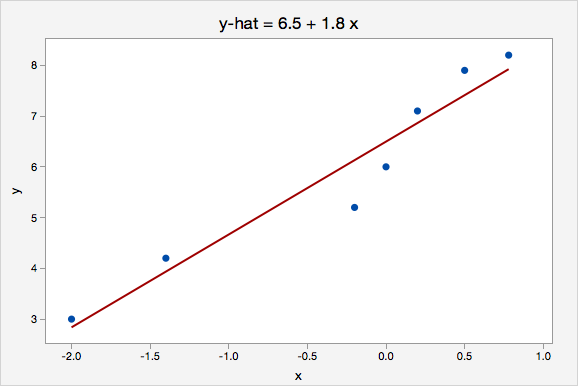Identify and interpret the y-intercept.

The y-intercept is 6.5. When $$x=0$$ the predicted value of y is 6.5.

Identify and interpret the slope.

The slope is 1.8. For every one unit increase in x, the predicted value of y increases by 1.8.

Compute and interpret the residual for the point (-0.2, 5.1).

The observed x value is -0.2 and the observed y value is 5.1.

The formula for the residual is $$e=y-\widehat{y}$$

We can compute $$\widehat{y}$$ using the regression equation that we have and $$x=-0.2$$

$$\widehat{y}=6.5+1.8(-0.2)=6.14$$

Given an x value of -0.2, we would predict this observation to have a y value of 6.14. In reality, they had a y value of 5.1. The residual is the difference between these two values.

$$e=y-\widehat{y}=5.1-6.14=-1.04$$

The residual for this observation is -1.04. This observation's y value is 1.04 less than predicted given their x value.

## Cautions

• Avoid extrapolation. This means that a regression line should not be used to make a prediction about someone from a population different from the one that the sample used to define the model was from.
• Make a scatterplot of your data before running a regression model to confirm that a linear relationship is reasonable. Simple linear regression constructs a straight line. If the relationship between x and y is not linear, then a linear model is not the most appropriate.
• Outliers can heavily influence a regression model. Recall the plots that we looked at when learning about correlation. The addition of one outlier can greatly change the line of best fit. In addition to examining a scatterplot for linearity, you should also be looking for outliers.

Later in the course, we will devote a week to correlation and regression.

# 3.4.3.1 - Minitab: SLR

3.4.3.1 - Minitab: SLR

## Minitab® – Simple Linear Regression

We previously created a scatterplot of quiz averages and final exam scores and observed a linear relationship. Here, we will use quiz scores to predict final exam scores.

1. Open the Minitab file: Exam.mwx (or Exam.csv)
2. Select Stat > Regression > Regression > Fit Regression Model...
3. Double click Final in the box on the left to insert it into the Responses (Y) box on the right
4. Double click Quiz_Average in the box on the left to insert it into the Continuous Predictors (X) box on the right
5. Click OK

This should result in the following output:

#### Regression Equation

Final = 12.1 + 0.751 Quiz_Average

#### Coefficients

Term Coef SE Coef T-Value P-Value VIF
Constant 12.1 11.9 1.01 0.3153
Quiz_Average 0.751 0.141 5.31 0.000 1.00

#### Model Summary

9.71152 37.04% 35.73% 29.82%

#### Analysis of Variance

Regression 1 2664 2663.66 28.24 0.000
Quiz_Average 1 2664 2663.66 28.24 0.000
Error 48 4527 94.31
Total 49 7191

#### Fits and Diagnostics for Unusual Observations

Obs Final Fit Resid Std Resid
11 49.00 70.50 -21.50 -2.25 R
40 80.00 61.22 18.78 2.03 R
47 37.00 59.51 -22.51 -2.46 R

R Large residual

#### Interpretation

In the output in the above example we are given a simple linear regression model of Final = 12.1 + 0.751 Quiz_Average

This means that the y-intercept is 12.1 and the slope is 0.751.

# 3.4.3.2 - Example: Interpreting Output

3.4.3.2 - Example: Interpreting Output

This example uses the "CAOSExam" dataset available from http://www.lock5stat.com/datapage.html.

CAOS stands for Comprehensive Assessment of Outcomes in a First Statistics course. It is a measure of students' statistical reasoning skills. Here we have data from 10 students who took the CAOS at the beginning (pre-test) and end (post-test) of a statistics course.

Research question: How can we use students' pre-test scores to predict their post-test scores?

Minitab was used to construct a simple linear regression model. The two pieces of output that we are going to interpret here are the regression equation and the scatterplot containing the regression line.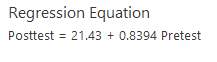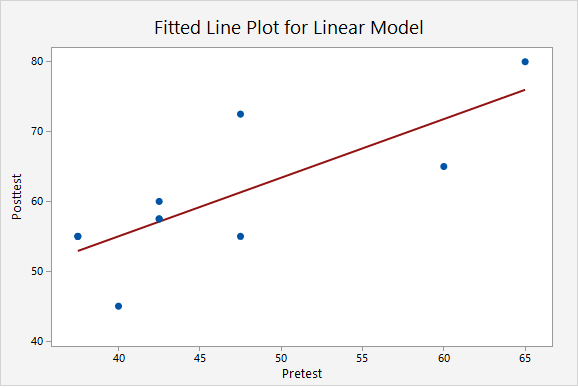Let's work through a few common questions.

What is the regression model?

The "regression model" refers to the regression equation. This is $$\widehat {posttest}=21.43 + 0.8394(Pretest)$$

Identify and interpret the slope.

The slope is 0.8394. For every one point increase in a student's pre-test score, their predicted post-test score increases by 0.8394 points.

Identify and interpret the y-intercept.

The y-intercept is 21.43. A student with a pre-test score of 0 would have a predicted post-test score of 21.43.  However, in this scenario, we should not actually use this model to predict the post-test score of someone who scored 0 on the pre-test because that would be extrapolation. This model should only be used to predict the post-test score of students from a comparable population whose pre-test scores were between approximately 35 and 65.

One student scored 60 on the pre-test and 65 on the post-test. Calculate and interpret that student's residual.

This student's observed x value was 60 and their observed y value was 65.

$$e=y- \widehat y$$

We have y.  We can compute $$\widehat y$$ using the x value and regression equation that we have.

$$\widehat y = 21.43 + 0.8394(60) = 71.794$$

$$e=65-71.794=-6.794$$

This student's residual is -6.794. They scored 6.794 points lower on the post-test than we predicted given their pre-test score.

# 3.5 - Relations between Multiple Variables

3.5 - Relations between Multiple Variables

Section 2.7 of the Lock5 textbook introduces you to some data visualizations that may be used with more than two variables. Here, we will look at a few examples of these data visualizations and how they can be interpreted.

# 3.5.1 - Scatterplot with Groups

3.5.1 - Scatterplot with Groups

A scatterplot with groups can be used to display the relationship between two quantitative variables and one categorical variable. Like the scatterplot that you learned about earlier, the quantitative variables are shown on the x- and y-axes. Now, the different levels of the categorical variable are communicated using different colored points or different shaped points.

## Minitab® – Scatterplots with Groups

This example will use the Palmer penguins dataset:

To create a scatterplot with groups in Minitab:

1. Open the data file in Minitab
2. From the tool bar, select Graph > Scatterplot > One Y Variable > Groups Overlaid
3. Double click the variable body_mass_g in the box on the left to insert it into the Y variable box on the right
4. Double click the variable flipper_length_mm in the box on the left to insert it into the X variable box on the right
5. Double click the variable species in the box on the left to insert it into the Group variables box on the right
6. Click OK

This should result in the following scatterplot with groups:

The scatterplot above shows the relationship between flipper length (in millimeters) and body mass (in grams) while noting which penguins were adelie, chipstrap, and gentoo by using markers that are different colors and shapes. Overall, there is a strong positive relationship between flipper length and body mass in this sample. Gentoo penguins tend to be larger than adelie or chipstrap penguins both in terms of their body mass and their flipper length.

Video Walkthrough

# 3.5.2 - Bubble Plots

3.5.2 - Bubble Plots

A bubble plot can be used to display data concerning three quantitative variables at a time and a categorical grouping variable.  In the example below, three variables are displayed: one on the $$x$$-axis, one on the $$y$$-axis, and one as the size of the bubbles. In Figures 2.75 and 2.76 in your textbook, four variables are displayed: quantitative variables are represented on the x-axis, y-axis, and as the size of the bubbles; a categorical variable is represented by the color of the bubbles.

## Example: Height, Weight, & Days Exercising

The plot below was made using the statistical software R. Data were collected from World Campus students. They were asked for their heights, weights, and how many days per week they exercised. Researchers believed that there would be a linear relationship between height and weight overall but that number of days exercised would also be a factor. In this plot height (in inches) is on the $$x$$-axis, weight (in pounds) is on the $$y$$-axis, and the size of each bubble is determined by the number of days per week that the individual exercised.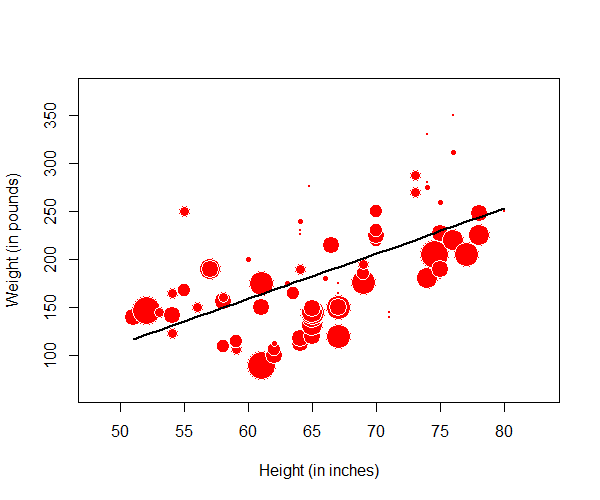Larger bubbles signify more days per week exercising. From this plot, we can see that there is a positive linear relationship between height and weight. We can also see that many of the larger bubbles (i.e., people who exercise more) tend to fall below the line of best fit and more of the smaller bubbles are above the line. In other words, people who spend more time at the gym have larger negative residuals. This means that for their height, they weigh less than predicted given a model that uses only height to predict weight.

## Example: Air Quality in New YorkSource: http://t-redactyl.io/blog/2016/02/creating-plots-in-r-using-ggplot2-part-6-weighted-scatterplots.html

The bubble plot above displays data for three quantitative variables plus a categorical variable. The x axis represents the day of the month and the y axis represents a measure of the air quality. The size of each bubble is the wind speed on that day. And, the color of the bubble represents the month.

It looks like the pink bubbles, particularly starting on the sixth of the month, tend to be lower than the blue and green. This means that air pollution tends to be lower in September compared to July and August. The larger bubbles tend to be lower, representing lower air pollution on windier days.

# 3.5.3 - Time Series Plot

3.5.3 - Time Series Plot

time series plot displays time on the $$x$$-axis and a quantitative response variable on the $$y$$-axis. Minitab will construct time series plots (Graphs > Time Series Plots) and will conduct time series analyses which are covered in upper-level statistics courses. Here, you should be able to interpret a time series plot.

## Example: Deaths by Horsekicks

Source: https://commons.wikimedia.org/wiki/File:R-horsekick_totals-by_year.svg

The time series plot above of "Deaths by horsekick in Prussian cavalry corps, 1875-94" displays the number of annual deaths in each of these 20 years. In other words, one quantitative variable is examined over time.

## Example: Changes in Religious Affiliations

Source: Duncan Keith: https://commons.wikimedia.org/wiki/File:Bsa-religion-question-1983-2006.svg

The time series plot above presents data from the British Social Attitudes Survey concerning religious affiliation. Here, percentages are compared for three different groups over time. We can see that the proportion of individuals who identify as Christian decreased during this time while the proportion who have no religion is increasing and the proportion of other non-Christians has stayed relatively steady and low.

# 3.6 - Lesson 3 Summary

3.6 - Lesson 3 Summary

## Objectives

Upon successful completion of this lesson, you will be able to:

• Construct and interpret a boxplot and side-by-side boxplots
• Use the IQR method to identify outliers
• Construct and interpret histograms with groups and dotplots with groups
• Construct and interpret a scatterplot
• Compute and interpret a correlation
• Construct and interpret a simple linear regression model (i.e., one explanatory and one response variable)
• Compute and interpret residuals from a simple linear regression model
• Interpret plots of more than two variables
• Select an appropriate graphical representation for a given scenario

* All graph construction and computations in this lesson will be conducted in Minitab

In Lessons 2 and Lesson 3 you learned about describing data. We have stressed the importance of being able to distinguish between categorical and quantitative variables. The summary statistics that you compute and the visual representations that you create are dependent on the number and types of variables that you are working with.

Before moving on to Lesson 4, you should check your understanding of the learning objectives above and review the objectives from Lessons 1 and Lesson 2 as well. These first three lessons will serve as a foundation for the rest of the course.

  Link ↥ Has Tooltip/Popover Toggleable Visibility